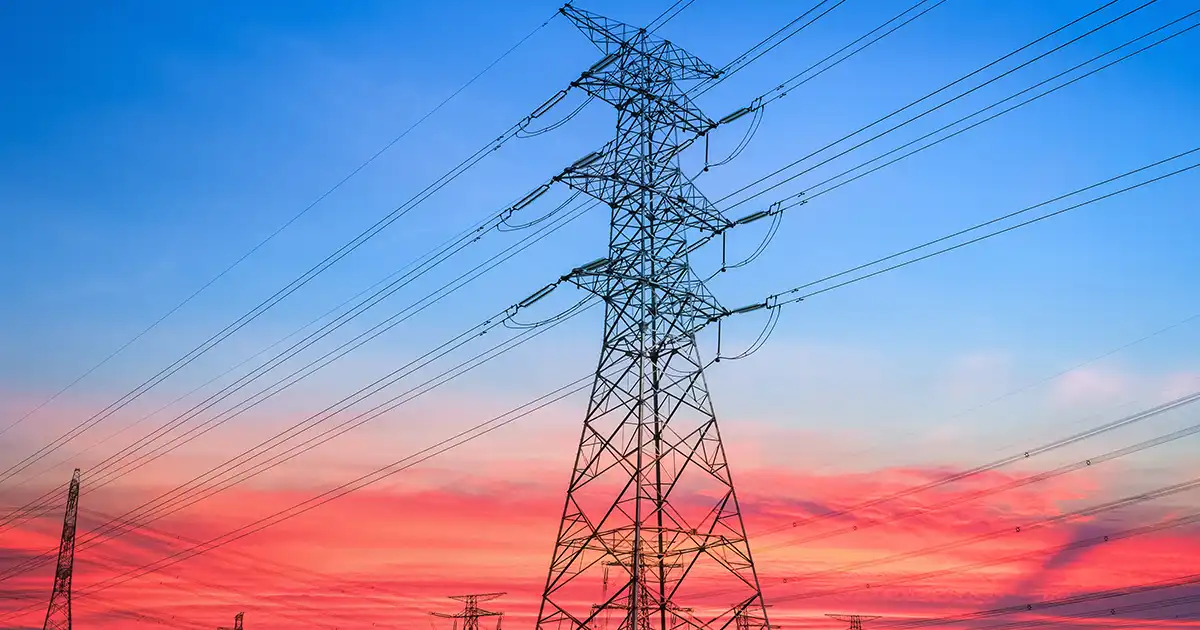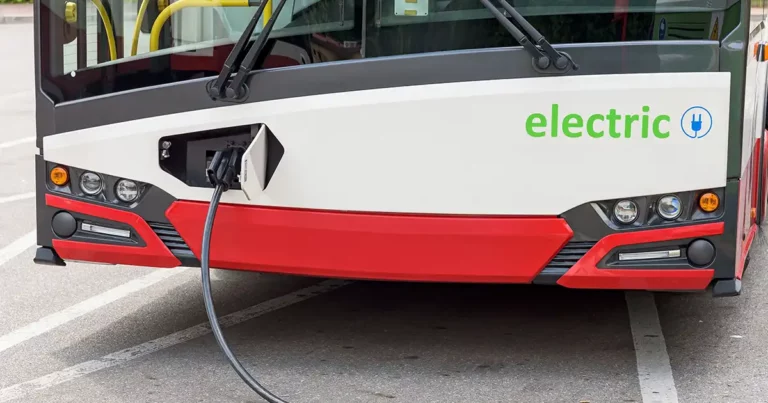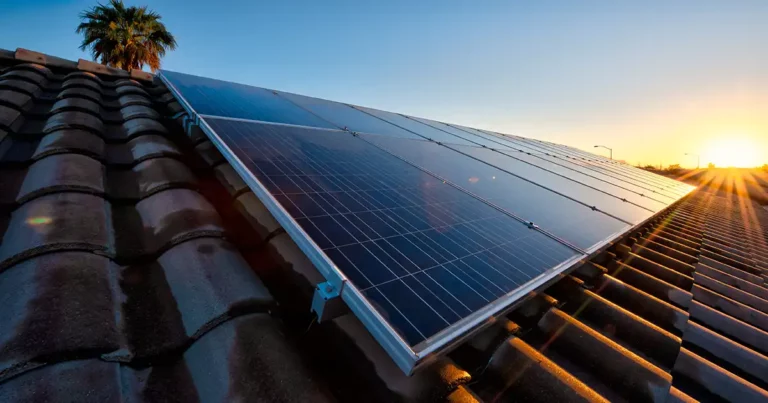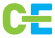# Basics of Electricity with Practical Examples

Introduction:

Electricity, a fundamental force shaping our modern world, is often considered mysterious and complex. In this comprehensive guide, we will demystify the basics of electricity, unraveling the intricacies of electric circuits, voltage, current, resistance, and more. Through real-life examples and calculations, we aim to provide you with a clear understanding of how electricity works, from the flow of electrons to the brilliance of a light bulb. Let’s embark on a journey to comprehend the essentials of electricity and witness its wonders through practical calculations.

Understanding Electric Circuits

Electric Circuit Fundamentals:

An electric circuit is a closed loop through which electric current flows. It consists of various components such as conductors, resistors, and power sources that work together to facilitate the flow of electricity.

Voltage (Measured in Volts):

Voltage is the force that drives the flow of electric current. It is measured in volts (V) and represents the electric potential difference between two points in a circuit.

Current (Flow of Electricity):

Electric current is the flow of electric charge. In a circuit, it is the movement of electrons through a conductor. Current is measured in amperes (A).

Calculating Voltage, Current, and Resistance

Ohm’s Law:

Ohm’s Law is a fundamental principle that relates voltage, current, and resistance. The formula is V = I * R, where V is voltage, I is current, and R is resistance (measured in ohms).

Example Calculation – Lighting up a Room:

Consider a scenario where you have a room with three light bulbs connected in series. Each bulb has a resistance of 4 ohms. If the voltage across the entire circuit is 12 volts, we can use Ohm’s Law to calculate the total current flowing through the circuit.

Voltage (V) = 12 volts

Resistance per bulb (R) = 4 ohms

Total resistance in the circuit (R_total) = 3 * 4 ohms = 12 ohms

Using Ohm’s Law: I = V / R

Current (I) = 12 V / 12 ohms = 1 A

Now, you know that 1 ampere of current is flowing through the circuit, illuminating the room with the connected light bulbs.

The Flow of Electrons: A Dance of Charges

Positive and Negative Charges:

Atoms consist of positively charged protons and negatively charged electrons. In a conductor, such as a copper wire, electrons are loosely bound and can move freely.

Flow of Electricity:

When a circuit is closed, electrons move from the negatively charged terminal of the power source (like a battery) through the conductor to the positively charged terminal, creating an electric current.

Electric Energy and Home Appliances

Electric Energy Conversion:

Electricity can be converted into other forms of energy. In various home appliances, electric energy is transformed into useful work, providing convenience in our daily lives.

Example – Cooking with an Electric Stove:

Imagine cooking dinner using an electric stove. The stove’s heating element converts electric energy into heat, allowing you to cook your favorite meals.

Calculating Energy Consumption:

Energy consumption is measured in kilowatt-hours (kWh). If you use a 1,000-watt microwave for 30 minutes, the energy consumption is 0.5 kWh (1000 watts * 0.5 hours / 1000).

The Role of Resistance: Impeding the Flow

Resistance in Circuits:

Resistance opposes the flow of electric current. Materials with high resistance require more voltage to maintain the same current flow.

Example – Heating Water with a Heater:

Consider an electric water heater with a resistance of 20 ohms. If the voltage is 120 volts, you can use Ohm’s Law to calculate the current flowing through the heater.

Voltage (V) = 120 volts

Resistance (R) = 20 ohms

Using Ohm’s Law: I = V / R

Current (I) = 120 V / 20 ohms = 6 A

The heater draws 6 amperes of current, converting electrical energy into heat to warm your water.

Electricity’s Journey Across Long Distances

Power Transmission:

Electricity can be transmitted over long distances using power lines. High voltage is used to minimize energy loss during transmission.

Imagine the journey of electricity from a power plant to your home. The transmission lines carry high-voltage electricity efficiently over long distances, ensuring you have a reliable power supply.

Conclusion: Illuminating the Path Forward

Electricity, once perceived as an enigma, reveals its secrets when approached with knowledge and curiosity. From the dance of electrons in a circuit to the brilliance of a light bulb and the convenience of home appliances, the basics of electricity form the foundation of our modern world. By grasping concepts like voltage, current, and resistance and applying them through practical calculations, you gain insights into the inner workings of this powerful force. As you navigate the complexities of electric circuits and witness the flow of electricity, remember that your newfound understanding illuminates the path to a world powered by knowledge and innovation.

## Check out more blogsA Comprehensive Guide to Solar Panels for Home Use...Solar Energy in Sports Stadiums in India: A Greener...Solar-Powered Public Transportation: Case Study in...The Rise of Mono PERC: A Game-Changer in Solar Energy...Solar Energy and the Fight Against Air Pollution Introduction...